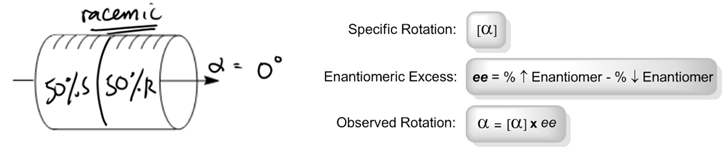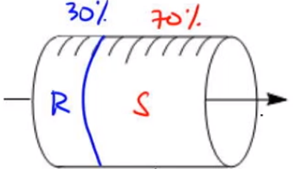Clutch Prep is now a part of Pearson
Ch. 5 - ChiralityWorksheetSee all chapters

# Enantiomeric Excess

See all sections
Sections
Constitutional Isomers vs. Stereoisomers
Chirality
Test 1: Plane of Symmetry
Test 2: Stereocenter Test
R and S Configuration
Enantiomers vs. Diastereomers
Atropisomers
Meso Compound
Test 3: Disubstituted Cycloalkanes
What is the Relationship Between Isomers?
Fischer Projection
R and S of Fischer Projections
Optical Activity
Enantiomeric Excess
Calculations with Enantiomeric Percentages
Non-Carbon Chiral Centers
Racemic Mixture
Achiral

Some more facts about optical activity:

• Specific rotation [α] is the rotation that 100% pure enantiomers produce.
• Opposite enantiomer = opposite rotation.
• Racemic: A perfect 1:1 ratio of enantiomers
• Scalemic: A non-1:1 ratio of enantiomers

Concept #1: How to calculate enantiomeric excess.

Transcript

Now what I want to do is I want to talk about enantiomeric excess, which is actually one of the most important concepts of optical activity.
Remember that I told you guys the specific rotation is the rotation that 100% enantiomers would produce. If I had a 100% enantiomer, let's say my specific rotation is 10 degrees, then I would get 10 degrees. It turns out that if I had the opposite enantiomer, meaning that this would be the R, but let's say I have the S enantiomer over here, then what would I get? I would just get the opposite rotation. Then that means that instead of getting a positive 10 rotation, maybe I'd get a negative 10, something like that. Basically, I'd do the same absolute value, just a different sign. That's the first thing that you guys need to know.
Second thing is that we can mix enantiomers. It doesn't always have to be a 100% pure solution. In fact, for most of these questions, it never will be. Your professor is going to mix it up and give you guys some kind of mixture and you're going to have to figure out what the optical activity is at the end of the mixture.
A perfect one-to-one ratio of enantiomers, meaning that I have 50% S and 50% R is called racemic. That's very important. That's such an important word for organic chemistry. You can never forget that. A non-one-to-one ratio, meaning that it's not pure but it's also not 50/50 is called scalemic. This one is not used quite as frequently, but it's still something that you should be aware of.
Now what I want to do is I want to take our polarimeter tubes, our sample tubes, and I want to do some experiments. We're just going to make up some numbers and we're just going to see what happens.
For this first one, as you can see, I have my S enantiomer and it has a specific rotation, that means the alpha in brackets, of 20. I want to know if I took 100%, if I had 100% of S in this tube, what would be the observed rotation? The answer is that the observed rotation would just be the same thing. It would be positive 20. I'm going to explain why in a second.
Then, the R enantiomer – let's say that my S enantiomer is positive 20, but let's say that I have 100% of my R enantiomer in here. Now I just mixed it up. Then what would that be? What that would give me is negative 20. Because it has an opposite configuration, so my observed would also be opposite.
Now what I want to do is let's say that I had in this one I had 50% of S and then I had 50% of R. What would be my observed rotation in that case? Just intuitively the way you can think of it is I have 50% rotating at 20 degrees to the right, I have 50% rotating at 20 degrees to the left, what would be the observed rotation? The observed rotation, or just the alpha symbol, not the alpha with the brackets, would be zero because they would cancel each other out. This is what we would call racemic. A racemic concentration is always going to have an optical activity of zero because it's going to perfectly cancel out. Does that make sense so far? Cool.
Now what I want to do is I want to talk about something called the enantiomeric excess. What the enantiomeric excess says is it's just basically you take your highest percentage enantiomer and subtract it from your lowest percentage enantiomer. You only have two enantiomers. You would take your highest minus your lowest and whatever you have at the end of that, that's going to be your enantiomeric excess. That's the amount that's actually optically active.
If we ever want to calculate observed rotation, it's actually really easy. All we do is say observed rotation, or alpha, equals the specific rotation, meaning the amount that molecule would theoretically produce at 100% times the enantiomeric excess, which is actually the optically active part because it's the part that isn't canceled out by anything else.Example #1: Calculate the ee and observed rotation for the following chiral mixture where S-enantiomer has [α] = +20.

Calculate the observed rotation when given the following ratio of enantiomers in solution.Example #2: Calculate the ee and observed rotation for the following chiral mixture where S-enantiomer has [α] = +20.

Transcript

All right, I'm going to go ahead and take myself out of this video so that you guys can see the full image. First of all, we had to figure out the enantiomeric excess. The way we figure that out is by saying, 70 minus 30% equals what percentage? 40% ee. That means that my 33 is 40%.
So what's my equation? My equation says that my observed rotation should be equal to my specific rotation which is still positive 20, times my ee, which is now going to be 40%. Now we don't really want to work with percentages if we're multiplying, so we would turn this into 0.4, which is just I'm turning my percentage into a decimal, which is very common.
Now I would just multiply these together. What I'm' going to get is that 20 times 0.4 is going to equal positive 8. My enantiomeric excess here should have been 40% and my observed rotation, instead of being positive 20 which is what it would be if it was 100, it's going to be positive 8.
How does that intuitively make sense? Actually, it makes a lot of sense. If you think about it, remember what I said about racemic, racemic isn't optically active. If I were to look at my tube as broken up into parts, what I would see is that the 70% is actually broken up into two parts. There's 30% here S that is perfectly canceled out by 30% R. What that means is that this entire area right here is racemic.
The racemic portion adds up to – I'm just going to put here racemic. The racemic portion adds up to what? It adds up to 60% because I have 30 and 30. That means that the only part of this that's optically active – let's put that back. That means that the only part of this that's optically active is the extra remaining S or the enantiomeric excess and that part equals 40%. That means that even though my S would love to rotate this positive 20, there's only 40% of it available that can actually rotate it because the other 30% is being canceled out by 30% of R.
What that means is that only 40% is functional and that's why it's only 40% of the total rotation that's happening. Out of the total rotation that I could have, only 40% of that is actually taking place, so it's positive 8.

We can also use these equations to calculate specific and observed rotation when other variables are given to us. Just plug the numbers in! Let's try a few.

Practice: When 0.200 g of lactose is dissolved in 10.0 ml of water and placed in a sample cell 10.0 cm in length, the observed rotation is +2°. Calculate the specific rotation of lactose.

Practice: Calculate the observed rotation of a chiral mixture that contains 65% (S)-stereoisomer where the [α] of pure (S)-stereoisomer = -118.

Practice: An optically pure (R)-stereoisomer of a molecule has a specific rotation of – 20°. What specific rotation would be observed for a mixture of the (R) and (S) stereoisomer where there is an enantiomeric excess equal to (S) 60%.